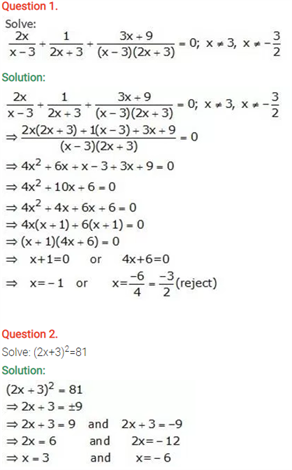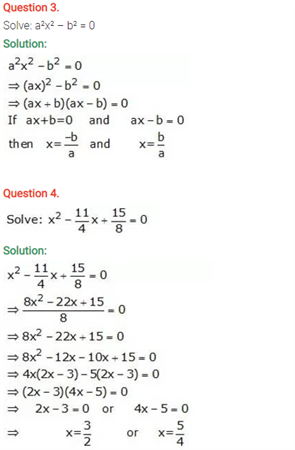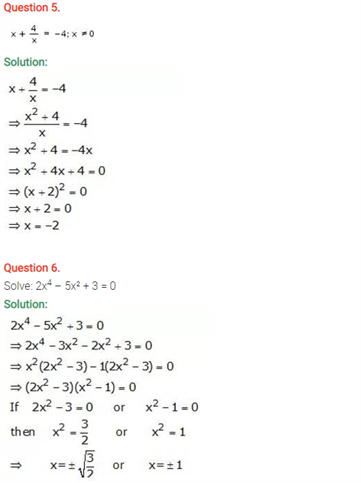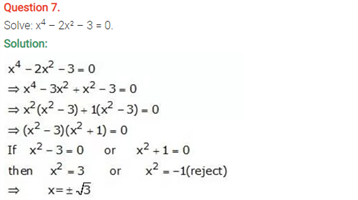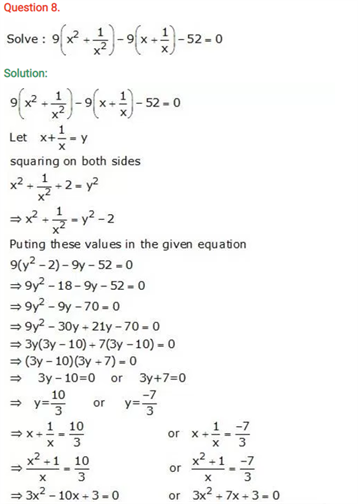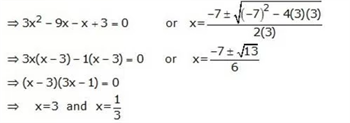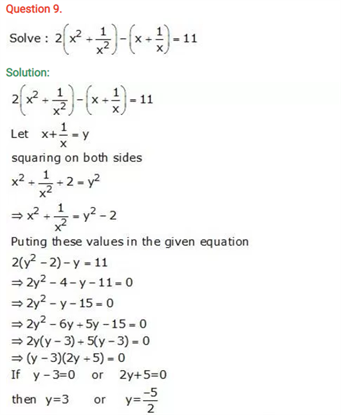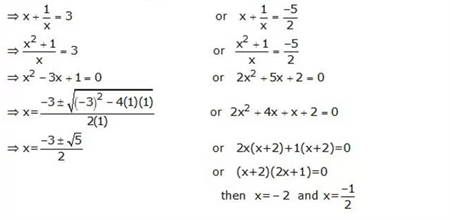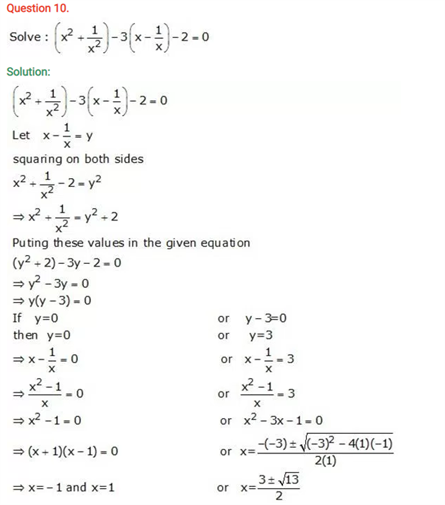# Selina Concise Maths Solution for ICSE Class 10 Chapter 5 Quadratic Equations

## Selina Class 10 Maths Solutions for Chapter 5

Quadratic equations are the equations that have the highest order as 2 which means it should have at least one squared term. The concept can be found in the ICSE Solutions For Class 10 Maths and are explained in a comprehensive manner. These questions will get you insights about quadratic equations. Lets us see the most basic definitions.

• A Quadratic equation can be written in the form fx2 +cx+d=e, where, f, c, d, and e are real numbers and f not be equal to 0.
• There are two ways to find the solutions, we can find what to multiply to make the Quadratic equation or we can complete the square.
• Or there is a third way, we could follow the quadratic formula and find x which is,

x = −b +/- √(b2 − 4ac)/2a

Students can find ICSE Class 10 Maths Selina Solutions Chapter 5 Quadratic Equations below: